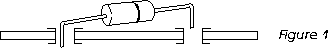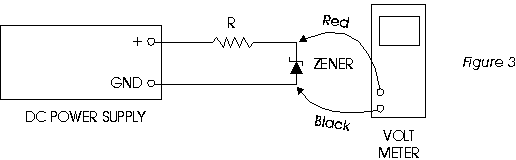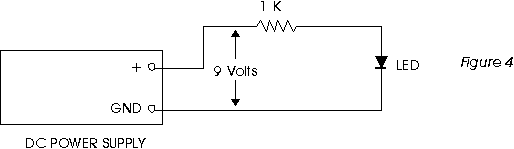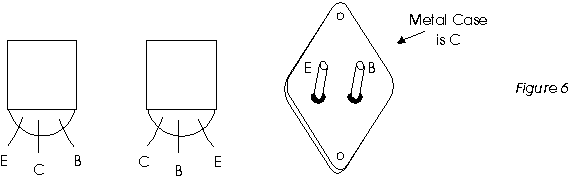● Tips and Tricks ●
 Electrical Tips and Tricks Testing Diodes and Transistors BACKGROUND One of the nice things about solid-state devices is that, under normal conditions, they rarely go bad. However, "rarely" is not the same as "never". And if conditions are not "normal", if an excessive voltage gets to a semiconductor, it can be damaged. In this article we will discuss how to test for a damaged transistor or diode. Device testing can be done at two levels: functional and parametric. A functional test determines whether or not the device works well enough for the intended use. A parametric test measures all device parameters to see if they meet the specified values. In the production of semiconductor devices, it is often the case that functional testing is done on all units while parametric testing is done on a small percentage of the units as test samples. For the most part, the performance of semiconductor devices does not deteriorate gradually over a period of time. Typically, transistors and diodes work well up to the point where they stop working completely, so all we will need to do is make a few simple functional tests. TESTING SILICON DIODES (NOT LED OR ZENER) To test a silicon diode such as a 1N914 or a 1N4001 all you need is an ohm-meter. If you are using an analog VOM type meter, set the meter to one of the lower ohms scales, say 0-2K, and measure the resistance of the diode both ways. If you get zero both ways, the diode is shorted. If you get INFINITY both ways, the diode is open. If you get INFINITY one way but some reading the other way (the value is not important) then the diode is good. If you use a digital multi-meter (DMM), then there should be a special setting on the Ohms range for testing diodes. Often the setting is marked with a diode symbol:Measure the diode resistance both ways. One way the meter should indicate an open circuit. The other way you should get a reading (often a reading around 600). That indicates the diode is good. If you measure an open circuit both ways, the diode is open. If you measure low resistance both ways, the diode is shorted. TESTING DIODES IN CIRCUIT The procedures described above assume the diode under test is not part of any circuit. If you are trying to test a diode that is on a circuit board or otherwise connected to other components, then you should disconnect one end of the diode. On a circuit board you can unsolder one end of the diode and lift it off the board. Make sure that you first disconnect all power going to the circuit before you disconnect the diode. After disconnecting one end, proceed as described above.KNOW POLARITY OF YOUR METER When set to measure resistance, both VOMs and DMMs apply voltage to the test leads. You should know which lead is positive. Don't assume the red lead is positive, it may not be. Use another meter set to measure DC volts on, say, the 20V scale and determine which lead of your Ohm-meter is positive. Another way is to take a diode you know is good and find which way you need to put the leads to get an Ohms reading. At that point, the positive lead is on the anode and negative lead is on the cathode (cathode is the banded end.)One reason to know the polarity of your meter is so you can determine which end of a diode is the cathode if the band has been removed. Also, as we will see below, you can use your Ohm-meter to tell an NPN transistor from a PNP if you know which meter lead is positive. TESTING ZENERS If you just want to know if a Zener diode has opened-up or shorted-out, then just test it as described above for standard diodes. if you want to measure its Zener voltage level, you will have to build a circuit as shown in Figure 3.The power supply voltage should be set to a value slightly higher than the Zener value. For example, for a 12 volt diode, the supply voltage should be about 15 volts. The value of the resistor R should limit the current to about a milliAmp. For example, using 15 volts with a 12 volt Zener, use a 3.3K resistor. The exact value is not critical. Once the circuit is built, just read the Zener voltage off the meter (if you read 0.6 volts, reverse the diode). NOTE: Any diode will become a Zener diode if you apply enough voltage to it. TESTING LEDS LEDs have a larger voltage-drop across them than regular diodes. Depending on the LED, the drop can be between 1.5 to 2.5 volts. If you have a DMM with a diode setting on the Ohms scale (see above), then you may be able to test an LED as you test a standard diode. The difference will be that the meter will read 1600 or 50 when the diode conducts instead of the 600 you read on a silicon diode. If you can't use your multi-meter, then build the circuit shown in Figure 4 and see if the LED light up.If the LED doesn't light, reverse polarity on the diode. If it still doesn't light, it's bad. (See Figure 4). TESTING ZENERS AND LEDS IN CIRCUIT To test a Zener or an LED while it is in a circuit, you just need a volt-meter. For a Zener, just measure the voltage across it. Using a VOM or a battery-operated DMM, put the black lead on the anode and the red lead on the cathode. You should read the Zener voltage. If you read zero volts, the Zener is shorted or the resistor feeding the Zener is open or not getting voltage. if you read a value higher than the Zener voltage, the Zener is open. For an LED that is supposed to be lit but isn't, use a VOM or battery-operated DMM to measure the voltage across it. If you measure more than 3 volts or so, the LED is open. TRANSISTORS As with diodes, it is usually the case that a transistor either works or it doesn't. So again we will be able to make a few simple tests with a meter to see if a transistor is good or bad. You can think of a transistor as two back-to-back diodes in one package as shown in Figure 5.Note that transistors come in two basic types: NPN and PNP. The letters C, B, E stand for COLLECTOR, BASE, EMITTER which are the names of the three leads which come out of a transistor. Transistors come in many different case styles, three of which are shown in Figure 6. It is important to know where C, B, E are for any given case.TESTING TRANSISTORS Assuming you know if the transistor is NPN or PNP, and assuming you know where B, C, and E are, then just test the B-C junction and the B-E junction as if they were standard diodes. if one of those junctions is a "bad diode", then the transistor is bad. Also, check the resistance from C to E using a higher Ohms scale (say, the 2 Meg scale). Be sure your fingers don't touch the metal test points or you will just measure your skin resistance. If the transistor is good, you should get an open-circuit reading from collector to emitter. NOTE: the above assumes silicon. With germanium transistors you may measure a high resistance from C to E. USING METER TO SEPARATE NPN FROM PNP If you have a transistor but you don't know if it is NPN or PNP, then you can find out which it is using your Ohm-meter if you know which lead of your meter is positive. Assuming you know where C, B, and E are on the transistor, do the following. Connect the positive lead of your Ohm-meter to the base. Touch the other lead of your meter to the collector. If you get a reading, the transistor is NPN. To verify, move the lead from the collector to the emitter and you should still get a reading. If your meter reads open-circuit, then connect the negative lead to the base and touch the positive lead to the collector. If you get a reading, then the transistor is PNP. Verify by measuring from base to emitter. THINGS TO WATCH FOR Some transistors have diodes from collector to emitter built into them. They will not read open-circuit when measuring resistance between C and E. Some transistors have resistors from base to emitter built into them. They will read that resistance when measuring Ohms B to E. Some transistors are Darlingtons. They have a higher reading base to emitter which may appear as an open on a VOM. CHECKING TRANSISTORS IN CIRCUIT With power disconnected from the circuit, you can try some of the above measurements on transistors that are in the circuit. However, your readings can be deceptive due to resistors and other components in the circuit. You can try disconnecting the base lead from the circuit before making measurements. Be sure to reconnect it after testing.